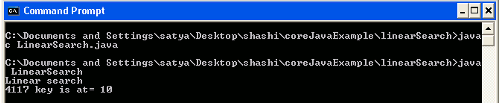# Linear search in java

In this section we will know, what is linear search and how linear works. Linear search is also known as "sequential search", by sequential it means it searches the element in sequence or in linear way.

In this section we will know, what is linear search and how linear works. Linear search is also known as "sequential search", by sequential it means it searches the element in sequence or in linear way.

## Linear search in java

In this section we will  know, what is linear search and how linear works. Linear search is also known as "sequential search",  by sequential it means it searches the element in sequence. Linear search is a searching mechanism which search  key element in sequential manner in array. Linear search is basically for small array but it will be a tedious job when it comes to larger size array. Time complexity in Linear search is more than any other searching algorithm. User have to enter the searching element, it will search the key element,  key is either a number or a string in array.

Example of Linear Search in Java:

```public class LinearSearch {
public static void main(String[] args)
{
System.out.println("Linear search");
int[] arr = {0,212, 1219, 781, 26, 124, 441, 127, 12,21,4117};
int i;
int key = 4117;
boolean flag=false;
for(i=0; i<arr.length; i++)
{
if(arr[i]==key){   // if searching element is same as key element then its return true in flag.
flag=true;
break;
}

}
if(flag)
{System.out.println(key + " key is at= "+i);}
else{System.out.println(key + " no not found");
}
}
}
```

In the above example, we have taken an array of fixed size. The method if(arr[i]==key) , if the searching element is same as key element then flag will return true else return false.

Output: After compiling and executing the above program.Download Source Code

Ads